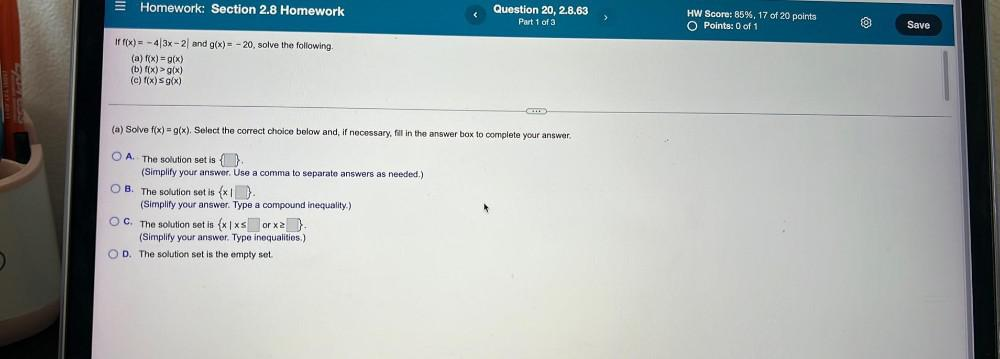Question:

# If f(x) = -4|3x - 2| and g(x) = -20, solve the following. (a) f(x) = g(x) (b) f(x) >g(x) (c) f(x) = g(x) (a) Solve f(x) = g(x). Select the correct choice below and, if necessary, fill in the answer boIf f(x) = -4|3x - 2| and g(x) = -20, solve the following. (a) f(x) = g(x) (b) f(x) >g(x) (c) f(x) = g(x) (a) Solve f(x) = g(x). Select the correct choice below and, if necessary, fill in the answer box to complete your answer. O A. The solution set is {□ }. (Simplify your answer. Use a comma to separate answers as needed.) O B. The solution set is {x| □} . (Simplify your answer. Type a compound inequality.) O C. The solution set is {x|x≤□ or x≥□}. (Simplify your answer. Type inequalities.) O D. The solution set is the empty set.